# How to Handle Time Series Data Sets

Time series can be challenging to work with as it requires special handling of the time dimension.
By Boris Delovski • Updated on Feb 4, 2023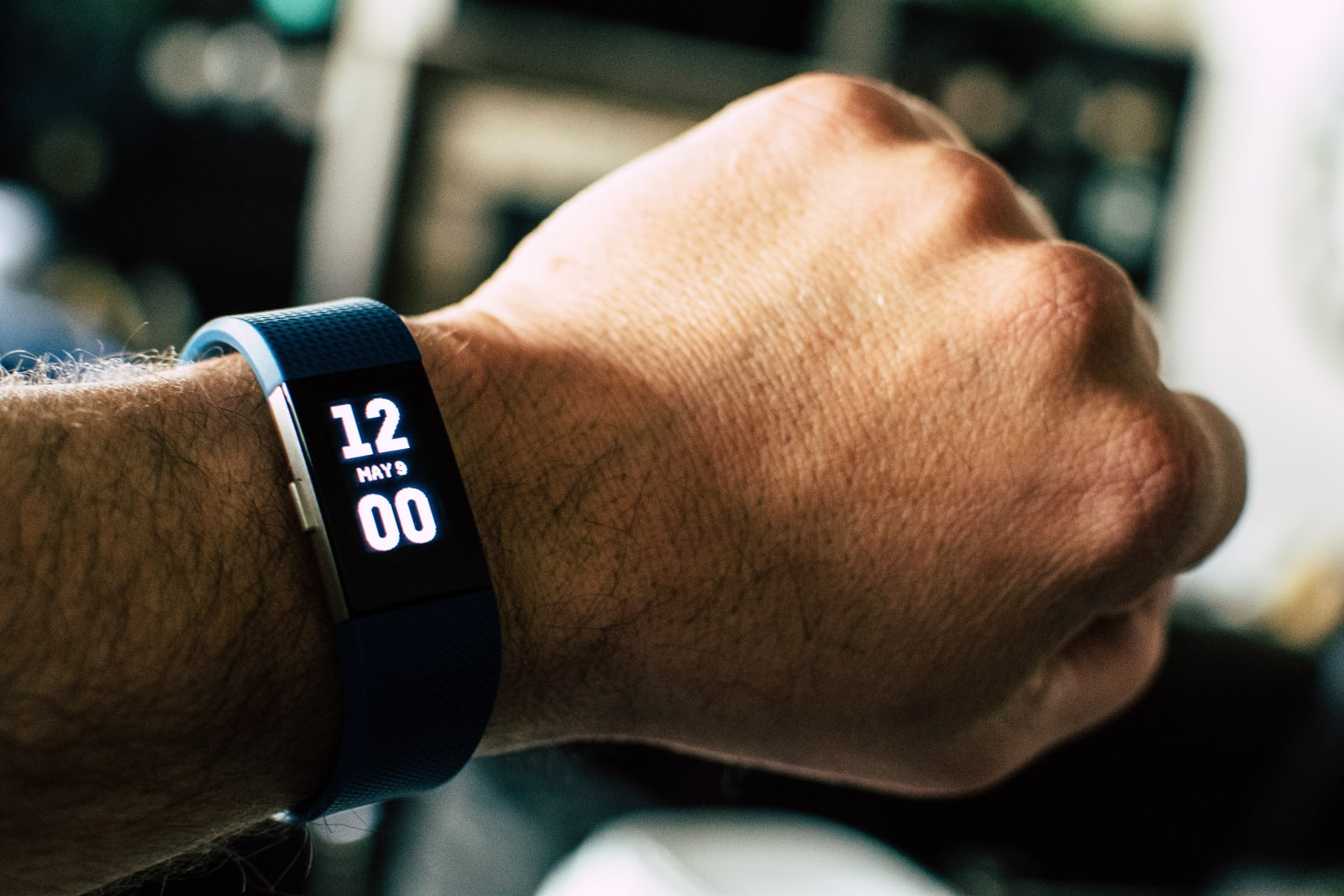Time is often used to track changes in data over time, such as stock prices, weather patterns, and traffic flow. When time is a valuable asset in your dataset, you’re working with a time series dataset. Time series can be challenging to work with as it requires special handling of the time dimension. However, with the right tools and techniques, it is possible to analyze and visualize time series data effectively. In this article, I will focus on explaining how you can represent time in Python by using the datetime module.

## How to Use the Datetime Module to Handle Time Series Datasets

Imagine you are analyzing the sales data of a retail shop. You want to find trends and patterns. You also want to compare sales from the same period across years and predict how well will the shop do in the next month. To accomplish those tasks, you must be able to sum and subtract time and filter your data to time slices. Those tasks become even more involved if you are dealing with an international franchise instead of a single store, where the time data comes from different time zones. The datetime module is a built-in Python module that allows you to handle such tasks. It allows you to store and manipulate date and time values in a variety of formats.

The datetime module also provides a range of functions and classes for working with dates and times, such as calculating the difference between two dates, formatting dates and times for display, and parsing strings into date and time objects. The datetime module eliminates the need to manually calculate the number of days in a month, or the number of seconds in a day. It even handles things like daylight savings time and leap years for you. This allows you to focus on the task at hand rather than worrying about the intricacies of date and time calculations.

The datetime module is extremely important because Pandas builds on the datetime library to offer its own set of datetime objects. Pandas is the most popular library for data processing and is the library almost everyone uses to work with time series data, so having a deeper understanding of how these datetime objects function can be very useful.

## What Are the Basics of the Datetime Module?

The main classes in the datetime module are the date, time, and datetime classes. All three are similarly constructed and have analogous methods and attributes. The first two incorporate information about a date and a time instance. The first uses the Gregorian calendar, and the second assumes that all days are the same. I will focus on the third as it is the most commonly used one and incorporates the two others.

Article continues below

### How to Create Datetime Objects

You instantiate the datetime class by passing the required year, month, and day arguments. If you want to, you can also include more details such as the hour, minute, second, microsecond, and even the time-zone. To start you can just instantiate the datetime class using the three main arguments.

For example:

``````# Import the datetime module
from datetime import datetime

# Create a datetime object for January 1, 2023, at 12:00 AM
new_year = datetime(2023, 1, 1)
print(new_year)``````

Running the code above will return the following output: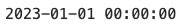As mentioned previously, you can also add information about the hour, second, etc., when creating a datetime object:

``````# Create a datetime object for January 2, 2023, at 8 hours, 23 minutes, and 32 seconds AM

work_time = datetime(2023, 1, 2, 8, 23, 32)
print(work_time)``````

Running the code above will return the following output: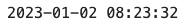One thing you may notice is that your code can quickly become hard to read. Therefore in most situations, it is better to explicitly define the values for your arguments:

``````# Create a datetime object for January 2, 2023, at 8 hours,
# 23 minutes, and 32 seconds AM
# Explicitly define values

work_time = datetime(
year=2023,
month=1,
day=2,
hour=8,
minute=23,
second=32)
print(work_time)``````

Running the code above will return the following output:While defining values manually is very useful, one of the most powerful features is the ability to create an object that uses the current date and time with microsecond precision using the now() function:

``````# Create a datetime object for the current instant

now = datetime.now()
print(now)``````

Running the code above will return the following output: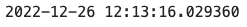## How to Extract Data From Datetime Objects

You can extract data from a datetime object by accessing its attributes. That way you can extract the day, month, year, etc., of a datetime object in a very efficient manner.

Here's an example:

``````# Create datetime object

dt = datetime(
year=2022,
month=1,
day=2,
hour=3,
minute=45,
second=46,
microsecond=789123)

# Extract parameters

print(f'dt.year = {dt.year}')
print(f'dt.month = {dt.month}')
print(f'dt.day = {dt.day}')
print(f'dt.hour = {dt.hour}')
print(f'dt.minute = {dt.minute}')
print(f'dt.second = {dt.second}')
print(f'dt.microsecond = {dt.microsecond}')``````

Running the code above will return the following output: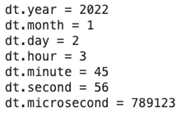To extract all the attributes at once, you can use the timetuple() method. This will not only return the values of the attributes mentioned above but will also return some other useful information. For instance, it will tell you which day of the week your object represents. The information itself is returned in the form of a tuple.

For example:

``````# Return the timetuple from dt

dt.timetuple()``````

Running the code above will return the following output: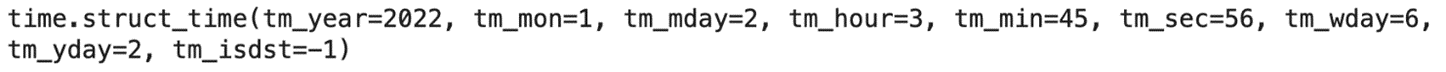By accessing attribute values, you can even manipulate them.

Suppose you got something wrong when instantiating or want to replace some information. This will happen often in practice, so it is very important to know how to fix this type of mistake. If you want to replace a certain value, you can create a new instance with the new value using the replace method:

``````# Create a new datetime object replacing the month in dt to April

dt1 = dt.replace(month=4)
print(dt1)``````

Running the code above will return the following output: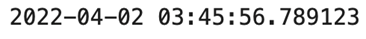## How to Create Timedelta Objects

A timedelta object represents an interval of time. You can use it to describe the difference between two points in time. For example,  if you go back to my example of being an owner of a retail store, then the time between placing an order and fulfilling an order would be represented with a timedelta object.

To create a timedelta object, you need to create an instance of the timedelta class. It represents the difference between two points in time using days, hours, minutes, etc. For example using the retail store, you can schedule a delivery 5 days from the order date by adding a timedelta to a datetime object.

``````# Import what we need

from datetime import timedelta

# Create datetime object

order_date = datetime(
year=2022,
month=1,
day=2,
hour=5,
minute=15)

# Calculate delivery date

delivery_date = order_date + timedelta(days=5)

# Display delivery date

print(delivery_date)``````

Running the code above will return the following output: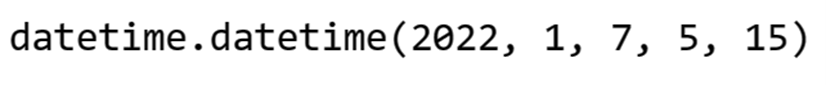You created a new datetime object by performing some arithmetic with datetime objects and timedelta objects.

You can perform many different operations: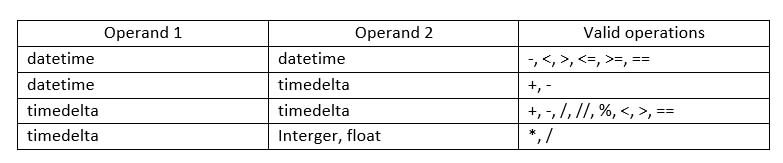The resulting object can be:

• A datetime object
• A timedelta object
• A boolean value
• An integer

The final result depends on the operands and on the operation that was performed; boolean values and integers appear rarely because they are created only when you compare or divide two timedelta objects.

I’ll demonstrate how you can get a boolean value:

``````# Define first order date and delivery date

order_date_1 = datetime(year=2022, month=1, day=2, hour=5, minute=15)
delivery_date_1 = datetime(year=2022, month=1, day=7, hour=5, minute=15)

# Define second order date and delivery date

order_date_2 = order_date_1
delivery_date_2 = datetime(year=2022, month=1, day=15, hour=5, minute=15)

# Define the two timedeltas

timedelta_1 = delivery_date_1 - order_date_1
timedelta_2 = delivery_date_2 - order_date_2

# Compare the two timedeltas

print(timedelta_2 <= timedelta_1)``````

Running the code above will return the following output:

## How to Use Parsing to Convert Data To and From a Datetime Object

Sometimes you can run into a very simple problem: when you were loading your data your time data was processed as a string. In that case, you must convert a string that represents an instance of time into a datetime object.

### How to Use the Strptime( ) Method

The simplest way to do this is using the strptime() method. Using a special type of string formatting, you can convert any string that represents a moment in time into a datetime object. When converting a string into a datetime object you use directives. They tell Python how it should treat a certain part of the string when converting the string into a datetime object.

Common directives are:

• %d, %m, %y: day, month, and year in a two digits format
•  %Y: four-digits year
•  %I, %H: hours in 12- and 24-hours clocks, respectively
•  %M, %S, %f: minutes, seconds, and microseconds
• %A, %B: day of the week and name of the month

You can find a complete list of directives here.

Here’s a demonstration on how to use directives:

``````# Create a string that represents a point in time

datetime_string = '07/21/22 15:55:26'

# Convert string into a datetime object

datetime_object = datetime.strptime(
datetime_string,
'%m/%d/%y %H:%M:%S')

# Display result

print(type(datetime_object))
print(datetime_object)  ``````

Running the code above will return the following output: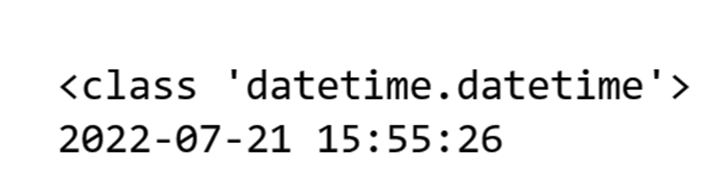### How to Use the Strftime( ) Method

You can also do the opposite: convert a datetime object into a string. The whole process is identical, just reversed; the same directives are used. The only difference is that you don't use the strptime() method but the strftime() method.

``````# Convert datetime object into a string

datetime_new_string = datetime.strftime(
datetime_object,
'%m/%d/%y %H:%M:%S')

# Display result

print(datetime_new_string)``````

Running the code above will return the following output:

### How to Use the Dateutil.parser Module

While you can use the datetime module to parse data, sometimes you need something more powerful. For parsing, you can use the dateutil.parser module. It allows you to parse your data from strings without needing to specify the exact format of the data stored in the string itself. You can parse data not only from ISO format but also from data that is formatted arbitrarily.

For example:

``````# Import the parse function

from dateutil.parser import parse

# Parse from ISO format

datetime_iso = parse('2013-01-07T00:00')
print(datetime_iso)

# Parse from a simple format

datetime_simple = parse('7th Jan, 2013')
print(datetime_simple)

# Parse from a less standard date

datetime_hard = parse('2013, January 7')
print(datetime_hard)``````

Running the code above will return the following output: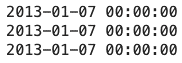In this article, I focused on the basics of using the datetime module. Many concepts such as what is a datetime object, how you manipulate them, how you format them, what timedelta objects are, etc., are the basic building blocks Pandas uses to handle time series data, so it is of the utmost importance to understand how they work. Once you understand how Pandas handles data that represents a series of moments in time and learn how to analyze time series data stored in Pandas, it becomes very easy; analyzing time series data with Pandas is the topic I am going to focus on in the subsequent article in this series of article on handling time series data with Python.# Boris Delovski

Boris is a data science trainer and consultant who is passionate about sharing his knowledge with others.

Before Edlitera, Boris applied his skills in several industries, including neuroimaging and metallurgy, using data science and deep learning to analyze images.